# NCERT Solutions for Class 10 Science Electricity Part 2## myCBSEguide App

CBSE, NCERT, JEE Main, NEET-UG, NDA, Exam Papers, Question Bank, NCERT Solutions, Exemplars, Revision Notes, Free Videos, MCQ Tests & more.

NCERT solutions for Class 10 Science Electricity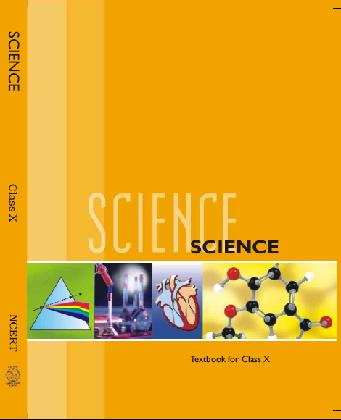## NCERT Class 10 Science Chapter wise Solutions

• 1 – Chemical Reactions and Equations
• 2 – Acids, Bases and Salts
• 3 – Metals and Non-metals
• 4 – Carbon and Its Compounds
• 5 – Periodic Classification of Elements
• 6 – Life Processes
• 7 – Control and Coordination
• 8 – How do Organisms Reproduce?
• 9 – Heredity and Evolution
• 10 – Light Reflection and Refraction
• 11 – Human Eye and Colourful World
• 12 – Electricity
• 13 – Magnetic Effects of Electric Current
• 14 – Sources of Energy
• 15 – Our Environment
• 16 – Management of Natural Resources

## NCERT Solutions for Class 10 Science Electricity Part 2

(a) 1/25

(b) 1/5

(c) 5

(d) 25

Ans. (d) 25

###### 2. Which of the following terms does not represent electrical power in a circuit?

(a) I2R

(b) IR2

(c) VI

(d) V2/R

Ans. (b) IR2

NCERT Solutions for Class 10 Science Electricity Part 2

###### 3. An electric bulb is rated 220 V and 100 W. When it is operated on 110 V, the power consumed will be:

(a) 100 W

(b) 75 W

(c) 50 W

(d) 25 W

Ans. (d) 25 W

NCERT Solutions for Class 10 Science Electricity Part 2

(a) 1:2

(b) 2:1

(c) 1:4

(d) 4:1

Ans. (c) 1:4

###### 5. How is a voltmeter connected in the circuit to measure the potential difference between two points?

Ans. A voltmeter is always connected in parallel to resistance across the point between which the potential difference is to be measured.

NCERT Solutions for Class 10 Science Electricity Part 2

###### 6. A copper wire has diameter 0.5 mm and resistivity of 1.6 x 10-8 m. what will be the length of this wire to make its resistance 10? How much does the resistance change if the diameter is doubled?

Ans. Diameter of wire (d) = 0.5 mm, resistivity (ρ) 1.6 x 10-8 Ωm, resistance (R) = 10 Ω.

R = ρL/A

L= πD2R/4ρ

= 22 x (5 x 10-4)2/ 7 x 4 x 1.6 x 10-8 = 122.5 m

If the diameter is doubled for given length of given material resistance is inversely proportional to the cross-section area of wire.

###### 7. The value of current I flowing in a given resistor for the corresponding values of potential difference V across the resistor are given below: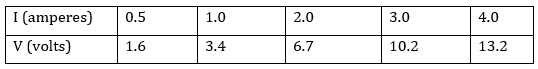###### Plot a graph between V and I and calculate the resistance of that resistor.

Ans. From the given data the I-V graph is a straight line as shown below: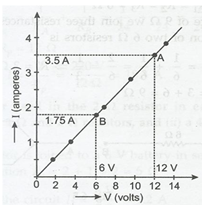Resistance of resistor (R) = VA-VB/1A-1B = 12 V – 6 V/ 3.6 A – 1.8 A

= 6V/ 1.8 A = 3.3 Ω

###### 8. When a 12 v battery is connected across an unknown resistor, there is a current of 2.5 mA in the circuit. Find the value of the resistance of the resistor.

Ans. Voltage of battery = V = 12 V, Current (I) = 2.5 mA = 2.5 x 10-3 A

Resistance (R) = V/I = 12V/ 2.5 x 10-3 A = 4800 Ω.

NCERT Solutions for Class 10 Science Electricity Part 2

###### 9. A battery of 9 V is connected in series with resistance of 0.2 Ω, 0.3 Ω, 0.4 Ω, 0.5 Ω and 12 Ω respectively. How much current would flow through the 12 resistor?

Ans. Potential difference (V) = 9 V.

Total resistance (R) = R1+ R2+ R3+R4 +R5

= 0.2 +0.3 + 0.5 + 0.5 + 12 = 13.4 Ω

Current in the circuit (I) = V/R = 9 V / 13. 4 Ω = 0.67 A.

In series circuit same current flows through all the resistance, hence current of 0.67 A will flow through 12 Ω resistor.

###### 10. How many 176 Ω resistors (in parallel) are required to carry 5 A on a 220 V line?

Ans. Let a resistor of 176 Ω are joined in parallel. Then their combined resistance (R)

1/R = 1/176 + 1/176 …… times = n/176 or R = 176/n Ω

It is given that V= 220 V and I = 5 A

R = V/I or 176/n = 220/5 = 44 Ω

n = 176/44 = 4, 4 resistors should be joined in parallel.

###### 11. Show how you would connect three resistors, each of resistance 6 Ω so that the combination has resistance of (i) 9 Ω (ii) 4 Ω.

Ans. It is given here that R1 = R2 = 6 Ω.

(i) To get net resistance of 9 Ω we should join three resistors as below: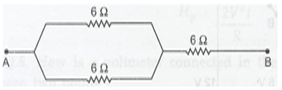(ii) To get 4 Ω net resistance we should join three resistors as below: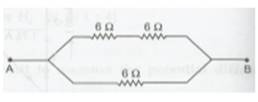NCERT Solutions for Class 10 Science Electricity Part 2

###### 12. Several electric bulbs designed to be used on a 220 V electric supply line, are rated 10 W. How many lamps can be connected in parallel with each other across the two wires of 220 V line if the maximum allowable current is 5 A?

Ans. Each bulb is rated as 10 W, 220 V, It draws a current (I) = P/V = 10 W/220 V

= 1/22 A.

As the maximum allowable current is 5 A and all lamps are connected in parallel, hence maximum number of bulbs joined in parallel with each other = 5 x 22 = 110.

NCERT Solutions for Class 10 Science Electricity Part 2

###### 13. A hot plate of an electric oven connected to a 220 V line has two resistance coils A and B. Each of 24 Ω resistances, which may be used separately, in series or in parallel. What are the currents in the three cases?

Ans. It is given that potential difference (V) = 220 V.

Resistance of coil (A) = Resistance of coil (B) = 24 Ω

(i) When either coil is used separately, the circuit (I) = V/R = 220 V/ 24 Ω

= 9.2 A.

(ii) When two coils are used in series total resistance (R)

= R1 + R2 = 24 +24 = 48 Ω

Current flowing (I) = V/ R = 220 V/ 48 Ω = 4.6 A.

(iii) When two coils are joined in parallel. Total resistance (R) = 1/24 + 1/24

= 2/24, R = 12 Ω.

Current (I) = V/R = 220 V / 12 Ω = 18.3 A.

###### 14. Compare the power used in the 2 Ω resistor in each of the following circuits:

(i) a 6-volt battery in series with 1 Ω and 2 Ω resistors and,

(ii) a 4 V battery in parallel with 12 Ω and Ω resistors.

Ans. (i) When a 2 Ω resistor is joined t a 6 V battery in series with 1 Ω and 2 Ω resistors. Total resistance (R) = 2 + 1 + 2 = 5 Ω.

Current (I) = 6 V/5 Ω = 1.2 A

Power used in 2 A resistor = I2R = 2.88 W

(ii) When 2 Ω resistor is joined to a 4 V battery in parallel with 12 Ω resistor and 2 Ω resistors, the current flowing in 2 Ω = 4 V/ 2 Ω = 2 A/.

Power used in 2 Ω resistor = I2R = 8 W

Ratio = 2.88/8 = 0.36: 1.

NCERT Solutions for Class 10 Science Electricity Part 2

###### 15. Two lamps, one rated 100 W at 220 V, and the other 60 W at 220 V are connected in parallel to electric mains supply. What current is drawn from the line if the supply voltage is 220 V?

Ans. Current drawn by 1st lamp rated 100 W at 220 V = P/V = 100/ 220 = 5/11 A.

Current drawn by 2nd lamp rated 60 W at 220 V = 60/220 = 3/11 A.

In parallel arrangement the total current = I1 +I2 = 3/11+ 5/11 = 8/11 = 0.73 A.

###### 16. Which uses more energy, a 250 W TV set in 1 hour, or a 1200 W toaster in 10 minutes?

Ans. Energy used by a TV set of power 250 W in 1 hour = P x t = 250 Wh.

Energy used by toaster of power 1200 W in 10 minute (10/60 h)

= P x t = 1200 W x 10/60 h = 200 Wh.

###### 17. An electric heater of resistance 8 draws 15 A from the service mains for 2 hours. Calculate the rate at which heat is developed in the heater.

Ans. Resistance of electric heater (R) = 8 Ω, current (I) = 15 A.

Rate at which heat developed in the heater = I2Rt/t = 15 x 15 x 8 = 1800 W.

NCERT Solutions for Class 10 Science Electricity Part 2

###### 18. Explain the following:

(a) Why is the tungsten used almost exclusively for filament of electric lamps?

(b) Why are the conductors of electric heating devices, such as bread-toasters and electric irons, made of an alloy rather than a pure metal?

(c) Why is the series arrangement not used for domestic circuits?

(d) How does the resistance of wire vary with its area of cross-section?

(e) Why are copper and aluminium wires usually employed for electric transmission?

Ans. (a) For filament of electric lamp we require a strong metal with high melting point. Tungsten is used exclusively for filament of electric lamps because its melting point is extremely high.

(b) Conductors of electric heating devices are made of an alloy rather than a pure metal due to high resistivity than pure metal and high melting point to avoid getting oxidized at high temperature.

(c) Series arrangement is not used for domestic circuits as current to all appliances remain same in spite of different resistance and every appliance cannot be switched on/ off independently.

(d) Resistance of a wire is inversely proportional to its cross-section area.

(e) Copper and aluminium wires are usually employed for electricity transmission because they are good conductor with low resistivity. They are ductile also to be drawn into thin wires.

## NCERT Solutions for Class 10 Science

NCERT Solutions Class 10 Science PDF (Download) Free from myCBSEguide app and myCBSEguide website. Ncert solution class 10 Science includes text book solutions from Book. NCERT Solutions for CBSE Class 10 Science have total 16 chapters. 10 Science NCERT Solutions in PDF for free Download on our website. Ncert Science class 10 solutions PDF and Science ncert class 10 PDF solutions with latest modifications and as per the latest CBSE syllabus are only available in myCBSEguide.

## CBSE app for Students

To download NCERT Solutions for class 10 Social Science, Computer Science, Home Science,Hindi ,English, Maths Science do check myCBSEguide app or website. myCBSEguide provides sample papers with solution, test papers for chapter-wise practice, NCERT solutions, NCERT Exemplar solutions, quick revision notes for ready reference, CBSE guess papers and CBSE important question papers. Sample Paper all are made available through the best app for CBSE students and myCBSEguide website.